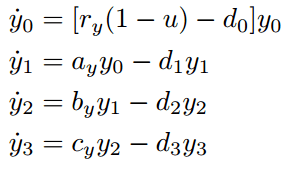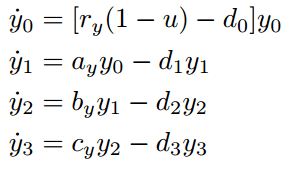# Solving a System of ODE for Steady State

Bewilder
I am trying to find the steady states in the ODE system. Assuming y0 = 2.5 * 10^5, I want to calculate y1, y2, y3 at the steady state. I do not understand how this would be possible, because only y0 is given and the following:

d0 = 0.003,
d1 = 0.008,
d2 = 0.05,
d3 = 1,
ry = 0.008,
ay = 1.6/100,
by = 10/750,
cy = 100,
u = 4 * 10^−8,
y0 = 2.5 * 10^5.

This is my ODE system:My task says: Find algebraically the steady state for the equations. Set y0 = 2.5 * 10^5 and calculate y1, y2, y3 based on the derived equations at the steady state.

Is it even possible to find the exact value of y1, y2, and y3 with the given information?

I have tried it in R, but it's impossible to get an answer.. How can I do this manually to get the solution for y1, y2, y3 at the steady state?

Code:
model <- function(t,x,params){
y0 <- x
y1 <- x
y2 <- x
y3 <- x
ry <- params
mu <- params
d0 <- params
ay <- params
d1 <- params
by <- params
d2 <- params
cy <- params
d3 <- params

m <- rep(0,4)
m = ((ry*(1-mu)) - d0) * y0
m = (ay * y0) - (d1 * y1)
m = (by * y1) - (d2 * y2)
m = (cy * y2) - (d3 * y3)

return(m)

}

x <- ode23(model, y0 = c(y0=250000, y1=y_1, y2=y_2, y3=y_3), t0=0,tf=400, params = c(0.008,4*10^-8,0.003,1.6/100,0.008,10/750,0.05,100,1))

Homework Helper
Dearly Missed
I am trying to find the steady states in the ODE system. Assuming y0 = 2.5 * 10^5, I want to calculate y1, y2, y3 at the steady state. I do not understand how this would be possible, because only y0 is given and the following:

d0 = 0.003,
d1 = 0.008,
d2 = 0.05,
d3 = 1,
ry = 0.008,
ay = 1.6/100,
by = 10/750,
cy = 100,
u = 4 * 10^−8,
y0 = 2.5 * 10^5.

This is my ODE system:My task says: Find algebraically the steady state for the equations. Set y0 = 2.5 * 10^5 and calculate y1, y2, y3 based on the derived equations at the steady state.

Is it even possible to find the exact value of y1, y2, and y3 with the given information?

I have tried it in R, but it's impossible to get an answer.. How can I do this manually to get the solution for y1, y2, y3 at the steady state?

Code:
model <- function(t,x,params){
y0 <- x
y1 <- x
y2 <- x
y3 <- x
ry <- params
mu <- params
d0 <- params
ay <- params
d1 <- params
by <- params
d2 <- params
cy <- params
d3 <- params

m <- rep(0,4)
m = ((ry*(1-mu)) - d0) * y0
m = (ay * y0) - (d1 * y1)
m = (by * y1) - (d2 * y2)
m = (cy * y2) - (d3 * y3)

return(m)

}

x <- ode23(model, y0 = c(y0=250000, y1=y_1, y2=y_2, y3=y_3), t0=0,tf=400, params = c(0.008,4*10^-8,0.003,1.6/100,0.008,10/750,0.05,100,1))

If by steady-state you mean that ##\dot{y}_i = 0 ## for ##i = 0, \ldots ,3## then your sought-for conditions are impossible. The only way to have ##y_0 \neq 0## but ##\dot{y}_0 = 0## would be to have the coefficient ##r_y(1-u) - d_0 =0##, and that is not true for the given data.

Bewilder
If by steady-state you mean that ##\dot{y}_i = 0 ## for ##i = 0, \ldots ,3## then your sought-for conditions are impossible. The only way to have ##y_0 \neq 0## but ##\dot{y}_0 = 0## would be to have the coefficient ##r_y(1-u) - d_0 =0##, and that is not true for the given data.

That's what has confused me. I am setting the equations equal to zero but it does not work, but it should since this system comes from a paper and they have solved it there.

http://ped.fas.harvard.edu/files/ped/files/nature05b_0.pdf?m=1425933222

This is the paper and the equations are in page 6 of the PDF. When the steady states are found then the simulation is run for 400 days and the results are plotted in page 3, Figure 4.a. But when I plot my simulation for 400 days (after setting equations equal to zero to find the steady state) what I get as plots is completely different from those shown in the paper..